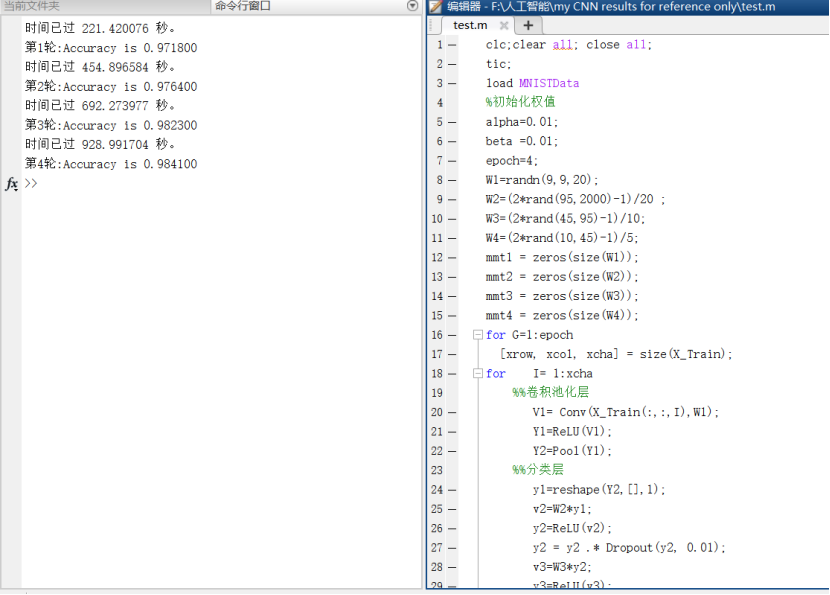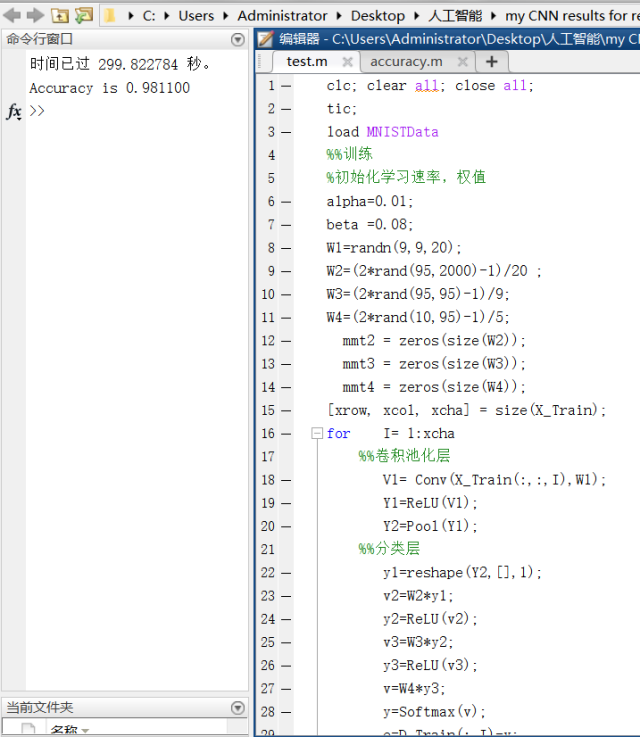2019-04-09 16:31:59 bayhax 阅读数 250
• ###### 云从科技：详解CNN-pFSMN模型以及在语音识别中的应用

近日，云从科技在语音识别技术上取得重大突破，在全球最大的开源语音识别数据集Librispeech上刷新了世界纪录，错词率（Worderrorrate，WER）降到了2.97%，将Librispeech的WER指标提升了25%，大幅刷新原先记录。本视频课程讲解了：大规模词汇连续语音识别，LVCSR前沿与最新进展，和CNN-pFSMN声学模型。

1122 人正在学习 去看看 CSDN讲师

# 写作目的

github链接: [https://github.com/bayhax/FlowerRecognize]

# python语音模块

## 语音转文字处理模块

apiai
pocketsphinx
SpeechRecognition
waston-developer-cloud

pip install SpeechRecognition

``````import speech_recognition as sr
sr.__version__
``````

``````import speech_recognition as sr
import logging
logging.basicConfig(level=logging.DEBUG)

while True:
#speech_recognition的核心是识别器类
r = sr.Recognizer()
#麦克风
mic = sr.Microphone()
logging.info('录音中')
with mic as source:
#去噪声
audio = r.listen(source)

logging.info('录音结束，识别中.....')
#print(test)
#如果不是空文件，返回的是一个包含列表的字典
if isinstance(test,list):
print("空")
else:
print(test['alternative']['transcript'])
print(type(test['alternative']['transcript']))
#print(type(test))
logging.info('end')

``````

## 文字转语音处理模块

pip install AipSpeech

``````# -*- coding: utf-8 -*-
#百度AI平台提供的语音库文件，playsound播放音频
from aip import AipSpeech
import shutil
#百度AI平台的ID秘钥等，APPID AK SK """
APP_ID = '你自己的ID'
API_KEY = '你自己的KEY'
SECRET_KEY = '你自己的秘钥'
#定义自己的初始化AipSpeech对象
myspeech = AipSpeech(APP_ID, API_KEY, SECRET_KEY)
num = 0
#要转换的文字信息
result  = myspeech.synthesis('二营长，把老子的意大利炮拿来', 'zh', 1, {
'spd':3,'pit':9,'vol': 6,'per':4
})
#如果识别正确，则返回语音的二进文件写入自己定义的audio文件中，错误则返回dict
#这里注意一下，with open打开写入文件用相对路劲有时候会出错，用绝对路径会相对好一些。
if not isinstance(result, dict):
with open(r'.\test\audio'+str(num)+'.mp3', 'wb') as f:
f.write(result)
#复制文件
shutil.copy(r'.\test\audio'+str(num)+'.mp3', r'.\testcopy\audio'+str(num)+'.mp3')
``````

## 播放音频文件模块

``````from pydub import AudioSegment
#要转换的文件的路径
filepath=".\\test\\audio0.mp3"
def trans_mp3_to_wav(filepath):
song = AudioSegment.from_mp3(filepath)
print("转换....")
#转换完的文件存储路径及格式
song.export(".\\testcopy\\test.wav", format="wav")

if __name__ == "__main__":
trans_mp3_to_wav(filepath)
``````

``````  def winCommand(*command):
buf = c_buffer(255)
command = ' '.join(command).encode(getfilesystemencoding())
errorCode = int(windll.winmm.mciSendStringA(command, buf, 254, 0))
if errorCode:
errorBuffer = c_buffer(255)
windll.winmm.mciGetErrorStringA(errorCode, errorBuffer, 254)
exceptionMessage = ('\n    Error ' + str(errorCode) + ' for command:'
'\n        ' + command.decode() +
'\n    ' + errorBuffer.value.decode())
raise PlaysoundException(exceptionMessage)
return buf.value

alias = 'playsound_' + str(random())
winCommand('open "' + sound + '" alias', alias)
winCommand('set', alias, 'time format milliseconds')
durationInMS = winCommand('status', alias, 'length')
winCommand('play', alias, 'from 0 to', durationInMS.decode())

if block:
sleep(float(durationInMS) / 1000.0)
``````

pip install playsound

``````from playsound import playsound
playsound("你自己的音频文件的路径")
``````

# 总结

2018-03-15 13:12:26 weixin_41376374 阅读数 80
• ###### 云从科技：详解CNN-pFSMN模型以及在语音识别中的应用

近日，云从科技在语音识别技术上取得重大突破，在全球最大的开源语音识别数据集Librispeech上刷新了世界纪录，错词率（Worderrorrate，WER）降到了2.97%，将Librispeech的WER指标提升了25%，大幅刷新原先记录。本视频课程讲解了：大规模词汇连续语音识别，LVCSR前沿与最新进展，和CNN-pFSMN声学模型。

1122 人正在学习 去看看 CSDN讲师

HMM，BP神经网络，GMM，DNN，RNN，CNN，LTSM，RNN-LTSM，DNN-HMM，CNN-HMM，GMM-HMM

2019-05-08 19:48:31 qq_41094877 阅读数 1606
• ###### 云从科技：详解CNN-pFSMN模型以及在语音识别中的应用

近日，云从科技在语音识别技术上取得重大突破，在全球最大的开源语音识别数据集Librispeech上刷新了世界纪录，错词率（Worderrorrate，WER）降到了2.97%，将Librispeech的WER指标提升了25%，大幅刷新原先记录。本视频课程讲解了：大规模词汇连续语音识别，LVCSR前沿与最新进展，和CNN-pFSMN声学模型。

1122 人正在学习 去看看 CSDN讲师

## 1.网络设计

1.1 CNN（特征提取网络＋分类网络）
随着深度学习的迅猛发展，其应用也越来越广泛，特别是在视觉识别、语音识别和自然语言处理等很多领域都表现出色。卷积神经网络（Convolutional Neural Network，CNN）作为深度学习中应用最广泛的网络模型之一，也得到了越来越多的关注和研究。事实上，CNN作为一项经典的机器学习算法，早在20世纪80年代就已被提出并展开一定的研究。但是，在当时硬件运算能力有限、缺乏有效训练数据等因素的影响下，人们难以训练不产生过拟合情形下的高性能深度卷积神经网络模型。所以，当时CNN的一个经典应用场景就是用于识别银行支票上的手写数字，并且已实际应用。伴随着计算机硬件和大数据技术的不断进步，人们也尝试开发不同的方法来解决深度CNN训练中所遇到的困难，特别是Kizhesky 等专家提出了一种经典的CNN架构，论证了深度结构在特征提取问题上的潜力，并在图像识别任务上取得了重大突破，热起了深度结构研究的浪潮。而卷积神经网络作为一种已经存在的、有一定应用案例的深度结构，也重新回到人们的视野中，得以进一步研究和应用。
而本次实验就是基于CNN实现的。
1.1.1基本架构
卷积神经网络基本架构包括特征抽取器和分类器。特征抽取器通常由若干个卷积层和池化层叠加构成，卷积和池化过程不断将特征图缩小，同时会导致特征图数量的增多。特征抽取器后面一般连接分类器，通常由一个多层感知机构成。特别地，在最后一个特征抽取器后面，将所有的特征图展开并排列成一个向量得到特征向量，并作为后层分类器的输入。
1.1.2卷积层
卷积运算的基本操作是将卷积核与图像的对应区域进行卷积得到一个值，通过在图像上不断移动卷积核和来计算卷积值，进而完成对整幅图像的卷积运算。在卷积神经网络中，卷积层不仅涉及一般的图像卷积，还涉及深度和步长的概念。深度对应于同一个区域的神经元个数，即有几个卷积核对同一块区域进行卷积运算；步长对应于卷积核移动多少个像素，即前后距离的远近程度。
本次实验卷积层采用的是20个99的滤波器进行滤波，激活函数为ReLU函数。
1.1.2.1局部感知
人对外界的认知一般可以归纳为从局部到全局的过程，而图像的像素空间联系也是局部间的相关性强，远距离的相关性弱。因此，卷积神经网络的每个神经元实际上只需关注图像局部的感知，对图像全局的感知可通过更高层综合局部信息来获得，这也说明了卷积神经网络部分连通的思想。类似于生物学中的视觉系统结构，视觉皮层的神经元用于局部接收信息，即这些神经元只响应某些特定区域的刺激，呈现出部分连通的特点。
1.1.2.2参数共享
局部感知过程假设每个神经元都对应100个参数，共106个神经元，则参数共有100×106个，依然是一个很大的数字。如果这106个神经元的100个参数相等，那么参数个数就减少为100，即每个神经元用同样的卷积核执行卷积操作，这将大大降低运算量。因不论隐层的神经元个数有多少，两层间的连接只要100个参数，这也说明了参数共享的意义。
1.1.2.3多核卷积
如果10×10维数的卷积核都相同，那么只能提取图像的一种特征，局限性很明显。可以考虑通过增加卷积核来提高特征类别，例如选择16个不同的卷积核用于学习16种特征。其中，应用卷积核到图像执行卷积操作，可得到图像的不同特征，统称为特征图（Feature Map），所以16个不同的卷积核就有16个特征图，可以视作图像的不同通道。此时，卷积层包含10×10×16=1600个参数。
1.1.3池化层
从理论上来看，经卷积层得到特征集合，可直接用于训练分类器（例如经典的Softmax分类器），但这往往会带来巨大计算量的问题。通过计算图像局部区域上的某特定特征的平均值或最大值等来计算概要统计特征。这些概要统计特征相对于经卷积层计算得到的特征图，不仅达到了降维目的，同时还会提高调练效率，这种特征聚合的操作叫作池化（Pooling），本次实验采用的是2
2的平均池化。
1.1.4 特征提取网络
使用reshape函数将特征提取网络的矩阵转换为2000*1的列向量，然后是两个隐层节点，这两个隐层节点含分别都含有95个神经元，中间采用的都是ReLU激活函数。最后是10个输出节点，实现单热编码输出，使用的是Softmax激活函数。

## 2. 训练方法

Delata规则＋BP算法＋交叉熵代价函数＋SGD（随机梯度下降算法）＋动量算法
2.1程序实现
使用到的 MNIST包自行下载后，需要用到的函数
accuracy

``````function y = accuracy(W1,W2,W3,W4,X_Test,D_Test,epoch)
N=length(D_Test);
d_comp=zeros(1,N);
for  k=1:N
X=X_Test(:,:,k);
V1=Conv(X,W1);%自定义函数（不旋转，直接滤波）
Y1=ReLU(V1);
Y2=Pool(Y1);%自定义函数，2×2平均池化操作
y1=reshape(Y2,[],1);
v2=W2*y1;
y2=ReLU(v2);
v3=W3*y2;
y3=ReLU(v3);
v=W4*y3;
y=Softmax(v);
[~,i]=max(y);%找到y向量中的最大元素，i为其位置索引
d_comp(k)=i;%保存CNN的计算值（识别出的数字）
end
[~,d_true]=max(D_Test);%将单热编码变回相应数字，存入d true（1xN维向量）
acc=sum(d_comp==d_true);%统计正确识别的总数
fprintf('第%d轮:',epoch);
fprintf('Accuracy is %f\n',acc/N);%输出正确率
end
``````

Conv

``````    function y = Conv(x, W)
%     对一幅图片的卷积
%
%     [wrow, wcol, numFilters] = size(W);
%     [xrow, xcol, ~] = size(x);
%     y = zeros(xrow - wrow + 1, xcol - wcol + 1, numFilters);
%     for k = 1:numFilters
%         y(:, :, k) = conv2(x, rot90(W(:, :, k),2), 'valid');
%     end

[xrow, xcol, xcha] = size(x);
[wrow, wcol, wpage, numFilters] = size(W);
if xcha>1&&(xcha==wpage)
y = zeros(xrow - wrow + 1, xcol - wcol + 1, numFilters);
W = W(:,:,end:-1:1,:);
for i = 1:numFilters
for j = 1:wpage
W(:,:,j,i) = rot90(W(:,:,j,i),2);
end
y(:, :, i) = convn(x, W(:,:,:,i), 'valid');
end
else
y = zeros(xrow - wrow + 1, xcol - wcol + 1, wpage);
for k = 1:wpage
y(:, :, k) = conv2(x, rot90(W(:, :, k),2), 'valid');
end
end

end
``````

Dropout

``````function ym = Dropout(y, ratio)
[m, n] = size(y);
ym     = zeros(m, n);
num     = round(m*n*(1-ratio));
idx     = randperm(m*n, num);
ym(idx) = m*n / num;
end
``````

Pool

``````function y = Pool(x)
%
% 2x2 mean pooling
%
%
y=(x(1:2:end,1:2:end,:)+x(2:2:end,1:2:end,:)+x(1:2:end,2:2:end,:)+x(2:2:end,2:2:end,:))/4;
end
``````

ReLU

``````function y = ReLU(x)
y = max(0, x);
end

Softmax
function y = Softmax(x)
ex = exp(x);
y  = ex / sum(ex);
end
``````

test

``````clc;clear all; close all;
tic;
%初始化权值
alpha=0.01;
beta =0.01;
epoch=20;
W1=randn(9,9,20);
W2=(2*rand(95,2000)-1)/20 ;
W3=(2*rand(45,95)-1)/10;
W4=(2*rand(10,45)-1)/5;
mmt1 = zeros(size(W1));
mmt2 = zeros(size(W2));
mmt3 = zeros(size(W3));
mmt4 = zeros(size(W4));
for G=1:epoch
[xrow, xcol, xcha] = size(X_Train);
for    I= 1:xcha
%%卷积池化层
V1= Conv(X_Train(:,:,I),W1);
Y1=ReLU(V1);
Y2=Pool(Y1);
%%分类层
y1=reshape(Y2,[],1);
v2=W2*y1;
y2=ReLU(v2);
y2 = y2 .* Dropout(y2, 0.01);
v3=W3*y2;
y3=ReLU(v3);
y3 = y3 .* Dropout(y3, 0.01);
v=W4*y3;
y=Softmax(v);
e=D_Train(:,I)-y;
%%误差前向传播
delta=e;%交叉熵＋softmax
e3=W4'*delta;
delta3=(v3>0).*e3;
e2=W3'*delta3;
delta2=(v2>0).*e2;
e1=W2'*delta2;
E2=reshape(e1,size(Y2));
E1=zeros(size(Y1));E2_4=E2/4;
E1(1:2:end,1:2:end,:)=E2_4;
E1(1:2:end,2:2:end,:)=E2_4;
E1(2:2:end,1:2:end,:)=E2_4;
E1(2:2:end,2:2:end,:)=E2_4;
delta1=(V1>0).*E1;
%%更新权值
[a,b,c]=size(W1);
for t=1:c
dW1(:,:,t)=alpha* conv2(X_Train(:,:,I),rot90(delta1(:,:,t),2),'valid');
mmt1(:,:,t)= dW1 (:,:,t)+ beta*mmt1(:,:,t);
W1(:,:,t)=W1(:,:,t)+mmt1(:,:,t);
%         W1(:,:,t)=W1(:,:,t)+dW1(:,:,t);
end
dW4=alpha*delta*y3';
mmt4 = dW4 + beta*mmt4;
W4   = W4 + mmt4;
%        W4   = W4 +dW4;
dW3=alpha*delta3*y2';
mmt3 = dW3 + beta*mmt3;
W3   = W3 + mmt3;
%        W3   = W3 +dW3;
dW2=alpha*delta2*y1';
mmt2 = dW2 + beta*mmt2;
W2   = W2 + mmt2;
%        W2   = W2 +dW2;
end

toc
%%统计正确率的代码：
acc=accuracy(W1,W2,W3,W4,X_Test,D_Test,G);
end
``````

2.2代码解释
2.2.1加载数据

``````clc; clear all; close all;
tic;
``````

2.2.2根据网络结构初始化学习速率，权值，循环次数等

``````alpha=0.01;
beta =0.01;
epoch=2
W1=randn(9,9,20);
W2=(2*rand(95,2000)-1)/20;
W3=(2*rand(45,95)-1)/10;
W4=(2*rand(10,45)-1)/5;
mmt2 = zeros(size(W2));
mmt3 = zeros(size(W3));
mmt4 = zeros(size(W4));
for G=1:epoch
``````

2.2.3训练CNN网络

``````[xrow, xcol, xcha] = size(X_Train);
for    I= 1:xcha
%%卷积池化层
V1= Conv(X_Train(:,:,I),W1);
Y1=ReLU(V1);
Y2=Pool(Y1);
%%分类层 ＋ dropout
y1=reshape(Y2,[],1);
v2=W2*y1;
y2=ReLU(v2);
y2 = y2 .* Dropout(y2, 0.01);
v3=W3*y2;
y3=ReLU(v3);
y3 = y3 .* Dropout(y3, 0.01);
v=W4*y3;
y=Softmax(v);
e=D_Train(:,I)-y;
``````

BP算法＋Delta规则＋交叉熵代价函数
%误差前向传播（交叉熵＋softmax）

`````` delta=e;
e3=W4'*delta;
delta3=(v3>0).*e3;
e2=W3'*delta3;
delta2=(v2>0).*e2;
e1=W2'*delta2;
E2=reshape(e1,size(Y2));
E1=zeros(size(Y1));E2_4=E2/4;
E1(1:2:end,1:2:end,:)=E2_4;
E1(1:2:end,2:2:end,:)=E2_4;
E1(2:2:end,1:2:end,:)=E2_4;
E1(2:2:end,2:2:end,:)=E2_4;
delta1=(V1>0).*E1;
``````

2.2.5 动量算法＋SGD更新权值

``````            [a,b,c]=size(W1);
for t=1:c
dW1(:,:,t)=alpha* conv2(X_Train(:,:,I),rot90(delta1(:,:,t),2),'valid');
mmt1(:,:,t)= dW1 (:,:,t)+ beta*mmt1(:,:,t);
W1(:,:,t)=W1(:,:,t)+mmt1(:,:,t);
%         W1(:,:,t)=W1(:,:,t)+dW1(:,:,t);
end
dW4=alpha*delta*y3';
mmt4 = dW4 + beta*mmt4;
W4   = W4 + mmt4;
%        W4   = W4 +dW4;
dW3=alpha*delta3*y2';
mmt3 = dW3 + beta*mmt3;
W3   = W3 + mmt3;
%        W3   = W3 +dW3;
dW2=alpha*delta2*y1';
mmt2 = dW2 + beta*mmt2;
W2   = W2 + mmt2;
%        W2   = W2 +dW2;
end

toc
``````

2.2.6 评估训练效果
%%统计正确率的代码：

``````acc=accuracy(W1,W2,W3,W4,X_Test,D_Test);
end
``````

## 3.实验结果上图分别为四轮次和单轮的最佳运行效果，成功达到了要求。

## 4.实验结果分析

通过对网络结构，算法，权值的不断调整，获得了目前最好的结果（98%＋正确率）。通过对训练后的权值和初始化的权值对比，不断调整其参数，尽量让初始化权值大小和训练后的结果差不多大，这样的训练效果最好。在SGD,小批量，批量中，根据运行的时间和精度不断比较，最终还是选择SGD算法最优。而动量算法对准确率提升不大，但是提升了稳定性，也提高了更新速度。在特征提取网络中，从一个隐层变成两个隐层，对实验结果精确率也有所帮助。而学习速率和动量算法的步进速率的选取，只能通过一次次实验寻找合适的值。一轮训练效果不够理想，所以进行了多轮训练。对于dropout,多轮次训练中可以有效解决过拟合问题。我也尝试了20001000100*10的分类层，虽然不符合设计要求，可是我发现节点更多，训练效果会更好一些。最高跑过10轮，可以达到98.9%的准确率。

## 5.结论

*卷积神经网络在图像、视频、语音和文本处理中取得了较多突破。在这一次的设计中，通过对代码的查找，修改，理解，自己从底层了解了卷积神经网络的一些概念，算法，优化方法。而且我认为只有如此才能理解其他编程软件的框架的由来，每一步在做什么。当然，也通过这次设计熟悉掌握了卷积神经网络在matlab上的代码操作。简单实现这次目标检测后，我也深刻认识到他还需要我更进一步的研究。
首先，由于CNN层数变得越来越深，人们对大规模的有效数据和高性能的计算能力也提出了越来越多的要求。同时，传统的人工搜集标签数据要求投入大量的人力和物力，这也导致了成本的提升，所以，无监督式的CNN学习方式越来越重要。
其次，为了提高CNN训练速度，一般采用某些异步的SGD算法，通过CPU和GPU集群可以得到一定的效果，但同时对硬件配置提出了一定的要求。因此，开发高效可扩展的训练算法依然有重要的实际价值。此外，深度模型在训练过程中往往需要在较长时间内占据较多的内存空间，对运行环境也带来了较大的压力。因此，在保证准确度的情况下，如何降低复杂性并快速训练得到模型，也是重要的研究方向。
再次，CNN应用于不同的任务要面临的关键问题是如何选择合适的训练参数，例如学习率、卷积核大小、卷积和池化层数等，这要求较多的技术积累和经验总结。这些训练参数存在内部相关性，也为参数调整带来较高的成本。因此，在CNN架构的选择上，依然值得我们去深入研究。

语音识别cnn程序 相关内容

2019-07-10 17:03:27 weixin_43772246 阅读数 10
• ###### 云从科技：详解CNN-pFSMN模型以及在语音识别中的应用

近日，云从科技在语音识别技术上取得重大突破，在全球最大的开源语音识别数据集Librispeech上刷新了世界纪录，错词率（Worderrorrate，WER）降到了2.97%，将Librispeech的WER指标提升了25%，大幅刷新原先记录。本视频课程讲解了：大规模词汇连续语音识别，LVCSR前沿与最新进展，和CNN-pFSMN声学模型。

1122 人正在学习 去看看 CSDN讲师

2019.7.10
今天的任务是看懂CNN，并自己搭建一个可以跑起来的CNN

## 什么是CNN

CNN是Convolutional Neural Networks的缩写，简而言之就是一种神经网络，只是其中进行的运算是卷积运算。具体的算法我在这里也就不说了，其他文章中也有相应的图文说明，大家自行查看。

## CNN的写法与用法

以下是我如何从0写出一个能跑起来的程序：
1、首先，我使用的pycharm来运行的，其中我们需要的库文件是这样：

``````import numpy as np
import struct
from keras.models import Sequential
from keras.layers import Dense
from keras.layers import Dropout
from keras.datasets import mnist
import matplotlib.pyplot as plt
from keras.layers import Flatten
from keras.layers.convolutional import Convolution2D
from keras.layers.convolutional import MaxPooling2D
from keras.utils import np_utils
``````

2、CNN又几个部分组成：Convolution层，MaxPooling层，Dropout层，Flatten层，Dense层，我来一个一个介绍
（1）指明结构
（2）Convolution层
`model.add(Convolution2D(20,(5,5),padding='same',input_shape=(1,28,28),activation='relu'))`
(3)MaxPooling层
这一层主要是做池化的，池化就是把一个框里的特征提一些出来，池化有两种一种是平均池化一种是最大值池化，我用的是最大值池化，就是把框里的特征的最大值提出来`model.add(MaxPooling2D(pool_size=(2,2),padding='same'))`
上面的代码就是把池化的框设为2×2，然后也变为了same，这样省的出错。
（4）Dropout层

``````model.add(Dropout(0.2))
``````

上面的代码意思是说添加一个Dropout层，防止过拟合，0.2为选择遗忘率，把一些权重忘记，这样不会过拟合，有时候这样还会使结果变好呢。
（5）Flatten层
无脑`model.add(Flatten())`就行。
（6）Dense层

``````model.add(Dense(128,activation='relu'))
model.summary()
return model
``````

128和50指的是神经元的数量，目前我还不知道他们这些数字有什么关系，反正就这么写吧，就这样，一个神经网络模型就已经搭好了，理论上讲，使用`model.fit(X_train, y_train, validation_data=(X_test, y_test), nb_epoch=10, batch_size=128, verbose=2)`往里输入特征矩阵，就可以输出一些东西了，这个就叫做训练。

## 本文涉及的最终代码

``````num_classes = y_test.shape

# define a simple CNN model
def simple_cnn():
model=Sequential()
model.summary()
return model
# build the model
model = simple_cnn()
# Fit the model
model.fit(X_train, y_train, validation_data=(X_test, y_test), nb_epoch=10, batch_size=128, verbose=2)

# Final evaluation of the model
scores = model.evaluate(X_test, y_test, verbose=0)
print("CNN Error: %.2f%%" % (100-scores*100))
``````

就可以输出错误率了。

## 能跑的程序

我下载了一个mnist的手写数据集，解压后放在跟python文件一个目录下就可以用了

``````import numpy as np
import struct
from keras.models import Sequential
from keras.layers import Dense
from keras.layers import Dropout
from keras.datasets import mnist
import matplotlib.pyplot as plt
from keras.layers import Flatten
from keras.layers.convolutional import Convolution2D
from keras.layers.convolutional import MaxPooling2D
from keras.utils import np_utils

# 训练集文件
train_images_idx3_ubyte_file = 'D:/first_py/train-images.idx3-ubyte'
# 训练集标签文件
train_labels_idx1_ubyte_file = 'D:/first_py/train-labels.idx1-ubyte'
# 测试集文件
test_images_idx3_ubyte_file = 'D:/first_py/t10k-images.idx3-ubyte'
# 测试集标签文件
test_labels_idx1_ubyte_file = 'D:/first_py/t10k-labels.idx1-ubyte'

def decode_idx3_ubyte(idx3_ubyte_file):
"""
解析idx3文件的通用函数
:param idx3_ubyte_file: idx3文件路径
:return: 数据集
"""
# 读取二进制数据

# 解析文件头信息，依次为魔数、图片数量、每张图片高、每张图片宽
offset = 0
magic_number, num_images, num_rows, num_cols = struct.unpack_from(fmt_header, bin_data, offset)
print('魔数:%d, 图片数量: %d张, 图片大小: %d*%d' % (magic_number, num_images, num_rows, num_cols))

# 解析数据集
image_size = num_rows * num_cols
print(offset)
fmt_image = '>' + str(image_size) + 'B'  #图像数据像素值的类型为unsigned char型，对应的format格式为B。这里还有加上图像大小784，是为了读取784个B格式数据，如果没有则只会读取一个值（即一副图像中的一个像素值）
print(fmt_image,offset,struct.calcsize(fmt_image))
images = np.empty((num_images, num_rows, num_cols))
#plt.figure()
for i in range(num_images):
if (i + 1) % 10000 == 0:
print('已解析 %d' % (i + 1) + '张')
print(offset)
images[i] = np.array(struct.unpack_from(fmt_image, bin_data, offset)).reshape((num_rows, num_cols))
#print(images[i])
offset += struct.calcsize(fmt_image)
#        plt.imshow(images[i],'gray')
#        plt.pause(0.00001)
#        plt.show()
#plt.show()

return images

def decode_idx1_ubyte(idx1_ubyte_file):
"""
解析idx1文件的通用函数
:param idx1_ubyte_file: idx1文件路径
:return: 数据集
"""
# 读取二进制数据

# 解析文件头信息，依次为魔数和标签数
offset = 0
magic_number, num_images = struct.unpack_from(fmt_header, bin_data, offset)
print('魔数:%d, 图片数量: %d张' % (magic_number, num_images))

# 解析数据集
fmt_image = '>B'
labels = np.empty(num_images)
for i in range(num_images):
if (i + 1) % 10000 == 0:
print ('已解析 %d' % (i + 1) + '张')
labels[i] = struct.unpack_from(fmt_image, bin_data, offset)
offset += struct.calcsize(fmt_image)
return labels

"""
TRAINING SET IMAGE FILE (train-images-idx3-ubyte):
[offset] [type]          [value]          [description]
0000     32 bit integer  0x00000803(2051) magic number
0004     32 bit integer  60000            number of images
0008     32 bit integer  28               number of rows
0012     32 bit integer  28               number of columns
0016     unsigned byte   ??               pixel
0017     unsigned byte   ??               pixel
........
xxxx     unsigned byte   ??               pixel
Pixels are organized row-wise. Pixel values are 0 to 255. 0 means background (white), 255 means foreground (black).

:param idx_ubyte_file: idx文件路径
:return: n*row*col维np.array对象，n为图片数量
"""
return decode_idx3_ubyte(idx_ubyte_file)

"""
TRAINING SET LABEL FILE (train-labels-idx1-ubyte):
[offset] [type]          [value]          [description]
0000     32 bit integer  0x00000801(2049) magic number (MSB first)
0004     32 bit integer  60000            number of items
0008     unsigned byte   ??               label
0009     unsigned byte   ??               label
........
xxxx     unsigned byte   ??               label
The labels values are 0 to 9.

:param idx_ubyte_file: idx文件路径
:return: n*1维np.array对象，n为图片数量
"""
return decode_idx1_ubyte(idx_ubyte_file)

"""
TEST SET IMAGE FILE (t10k-images-idx3-ubyte):
[offset] [type]          [value]          [description]
0000     32 bit integer  0x00000803(2051) magic number
0004     32 bit integer  10000            number of images
0008     32 bit integer  28               number of rows
0012     32 bit integer  28               number of columns
0016     unsigned byte   ??               pixel
0017     unsigned byte   ??               pixel
........
xxxx     unsigned byte   ??               pixel
Pixels are organized row-wise. Pixel values are 0 to 255. 0 means background (white), 255 means foreground (black).

:param idx_ubyte_file: idx文件路径
:return: n*row*col维np.array对象，n为图片数量
"""
return decode_idx3_ubyte(idx_ubyte_file)

"""
TEST SET LABEL FILE (t10k-labels-idx1-ubyte):
[offset] [type]          [value]          [description]
0000     32 bit integer  0x00000801(2049) magic number (MSB first)
0004     32 bit integer  10000            number of items
0008     unsigned byte   ??               label
0009     unsigned byte   ??               label
........
xxxx     unsigned byte   ??               label
The labels values are 0 to 9.

:param idx_ubyte_file: idx文件路径
:return: n*1维np.array对象，n为图片数量
"""
return decode_idx1_ubyte(idx_ubyte_file)
seed = 7
np.random.seed(seed)
#加载数据
'''导入文件'''
# reshape to be [samples][channels][width][height]
X_train = X_train.reshape(X_train.shape, 1, 28, 28).astype('float32')
X_test = X_test.reshape(X_test.shape, 1, 28, 28).astype('float32')
#print(X_train.shape)
# normalize inputs from 0-255 to 0-1
X_train = X_train / 255
X_test = X_test / 255

# one hot encode outputs
y_train = np_utils.to_categorical(y_train)
y_test = np_utils.to_categorical(y_test)
num_classes = y_test.shape

# define a simple CNN model
def simple_cnn():
model=Sequential()
model.summary()
return model
# build the model
model = simple_cnn()
# Fit the model
model.fit(X_train, y_train, validation_data=(X_test, y_test), nb_epoch=10, batch_size=128, verbose=2)

# Final evaluation of the model
scores = model.evaluate(X_test, y_test, verbose=0)
print("CNN Error: %.2f%%" % (100-scores*100))
``````

语音识别cnn程序 相关内容

2017-10-16 23:22:50 ai100_ml 阅读数 255
• ###### 云从科技：详解CNN-pFSMN模型以及在语音识别中的应用

近日，云从科技在语音识别技术上取得重大突破，在全球最大的开源语音识别数据集Librispeech上刷新了世界纪录，错词率（Worderrorrate，WER）降到了2.97%，将Librispeech的WER指标提升了25%，大幅刷新原先记录。本视频课程讲解了：大规模词汇连续语音识别，LVCSR前沿与最新进展，和CNN-pFSMN声学模型。

1122 人正在学习 去看看 CSDN讲师

### AI100_机器学习日报 2017-09-21

@好东西传送门 出品,由@AI100运营， 过往目录 见http://geek.ai100.com.cn

订阅：关注微信公众号 AI100（ID：rgznai100，扫二维码），回复“机器学习日报”，加你进日报群本期话题有：

全部23 深度学习10 算法6 资源4 自然语言处理2 视觉2 语音1 会议活动1 经验总结1

用日报搜索找到以前分享的内容： http://ml.memect.com/search/

## 今日焦点 (5)

统计

《Ngram2vec: Learning Improved Word Representations from Ngram Co-occurrence Statistics》Z Zhao, T Liu, S Li, B Li, X Du [Renmin University of China & Beijing Normal University] (2017) http://t.cn/R0zMukO GitHub: https ://github .com/zhezhaoa/ngram2vec ​

Jeff Dean 行业动态

Jeff Dean两年AMA全盘点：26个关于谷歌大脑和机器学习未来的问题（上） http://t.cn/R0wjj0v

语音

【微信小程序语音识别服务搭建全过程解析（项目开源在github）】silk v3录音转olami语音识别和语义处理的api服务（ubuntu16.04服务器上实现） 重要的写在前面 重要事项一： 目前本文中提到的API已支持微信小程序录音文… 详戳→ http://t.cn/R0wZ31Q 作者→ (墨迹斑斑被占用) ​

深度学习

【NASA机器学习/深度学习应用】《NASA Perspectives on Deep Learning – insideHPC》by Nikunj Oza http://t.cn/R0zMkq4

## 最新动态

### 2017-09-21 (14)

视觉 王亮

【王亮：由AI Challenger漫谈数据集的重要性】首届“AI Challenger·全球AI挑战赛”日前开启，AI Challenger全球AI挑战赛的评委、中科院自动化所#模式识别#国家重点实验室副主任王亮博士，解答最常见的一些数据集相关的问题，王亮博士也向我们解释了图像领域经典数据集之所以经典的原因…#人工智能# ​

视觉 算法 强化学习

【金融、视觉领域之外，AI也成为游戏开发的颠覆性技术】“深度强化学习”在游戏开发中的运用尽管仍处于初期发展阶段，但是我们清楚地知道它将很可能成为一项颠覆性的游戏技术。http://t.cn/R0zRf4h

算法 资源 课程

【2017高斯过程与不确定性量化暑期学校(视频)】“Gaussian Process and Uncertainty Quantification Summer School, 2017” http://t.cn/R0zN5vr

深度学习 教育网站 可视化

【VAST’17最佳论文：TensorFlow深度模型图可视化】《Visualizing Dataflow Graphs of Deep Learning Models in TensorFlow》K Wongsuphasawat, D Smilkov, J Wexler, J Wilson… [University of Washington] (2017) http://t.cn/R0zC36E http://t.cn/R0zC0mH

深度学习 代码

【PyTorch实现的段向量(doc2vec)】’Paragraph Vectors – PyTorch implementation of Paragraph Vectors (doc2vec)’ by Nejc Ilenic GitHub: http://t.cn/R0zChMG

【自动机器学习：让AI系统选择最佳AI】《Automatic Machine Learning: Artificial Intelligence Systems Choosing the Best Artificial Intelligence | Tractica》 http://t.cn/R0z9B6j

算法 资源 Yaser Abu-Mostafa 课程

【加州理工学院：机器学习与数据挖据】实现真正的人工智能就必须教会机器“自己学习”，而数据则是机器学习研究领域的基础。本课程由加州理工开设，机器学习领域著名学者Yaser Abu-Mostafa主讲，讲解机器学习与数据挖掘的基础理论、算法与应用。 ​​​​ http://t.cn/R2MR0Rj

会议活动 资源 自然语言处理 PDF VLDB 会议

【Mison: A Fast JSON Parser for Data Analytics】微软研究院和匹兹堡大学论文《Mison：用于数据分析的快速JSON解析器》，PDF格式，下载链接：http://t.cn/R0zofMC

自然语言处理 行业动态 新闻

【谷歌推出云端自然语言处理功能：通过语言分析情绪】谷歌发布了两项云端自然语言处理功能，可以帮助第三方开发者组织内容，理解客户对企业、产品或地点的感受。自动归类是一项测试功能，可以自动对内容进行归类，帮助企业把具备共同特点的内容进行归档。 http://t.cn/R0vrAQ3

经验总结 深度学习 Adrian Rosebrock Python 经验

【AWS(Python)深度学习AMI镜像及使用指南】《Pre-configured Amazon AWS deep learning AMI with Python | PyImageSearch》by Adrian Rosebrock http://t.cn/R0zJ2j7 Reddit: http://t.cn/R0zJ2jh

算法 Greg Tam 集成学习 决策树

【决策树/随机森林深入解析】《Interpreting Decision Trees and Random Forests · Pivotal Engineering Journal》by Greg Tam http://t.cn/R0zxVH0

深度学习 资源 Chris Benson 视频

【Go语言深度学习】《Deep Learning with Go – YouTube》by Chris Benson http://t.cn/R0zxPAS

深度学习 算法 CRF Jiao Tong 论文 神经网络

《Empower Sequence Labeling with Task-Aware Neural Language Model》 [University of Illinois at Urbana-Champaign & University of Southern California & Shanghai Jiao Tong University & Facebook] (2017) http://t.cn/R0zMRIM GitHub: https ://github .com/LiyuanLucasLiu/LM-LSTM-CRF ​

算法 论文 神经网络

《A Probabilistic Framework for Nonlinearities in Stochastic Neural Networks》Q Su, X Liao, L Carin [Duke University] (2017) http://t.cn/R0zMOyg

语音识别cnn程序 相关内容

阅读数 2932

博文 来自： zhou_xw6511

阅读数 266

博文 来自： weixin_41697507

阅读数 18278

博文 来自： zhoubl668
没有更多推荐了，返回首页